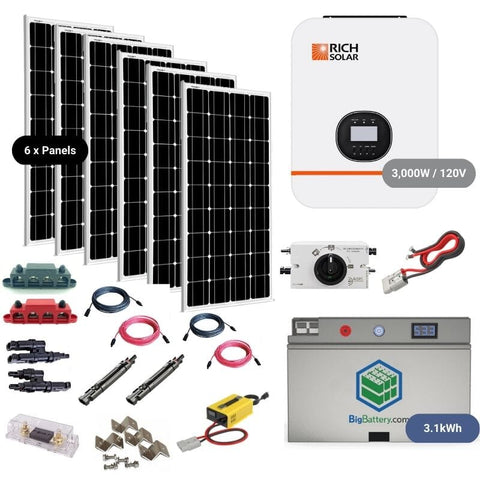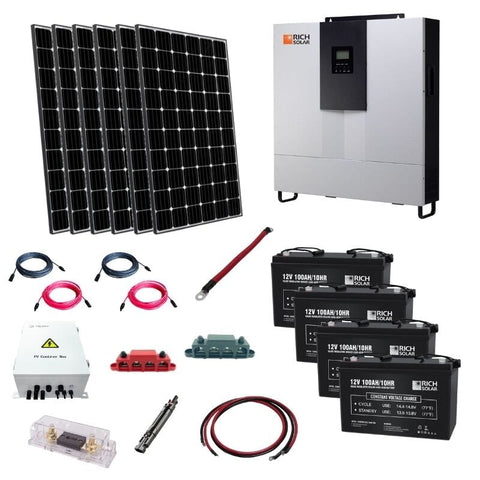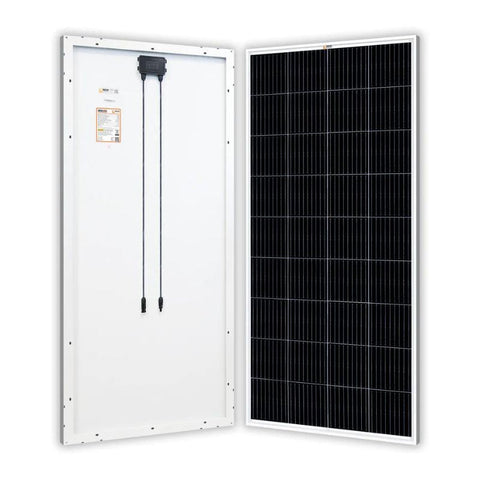Convert Volts to Amps (Electrical Conversions & Guide) - ShopSolar.com

# Convert Volts to Amps

It’s difficult not to get swept up in all the excitement around sustainable power and all the benefits it can provide for both you and the planet. However, to get the most from your solar panel setup, it helps to know how to do some basic electrical calculations, including converting volts to amps.

This doesn’t mean you have to go back to school though. There are loads of useful resources on our blog to take you through every step of the way to unpacking the various equations you need to familiarise yourself with.

You’ll even be able to make sense of your power bill when you know how to convert amp hours to kWh or convert mW to kWh.

Today, we’re going to go over how to convert volts to amps and vice versa. We’re also going to cover why it’s so important to be able to do this and exactly when it will come in handy.

## Why Do You Need to Convert Volts to Amps?

Determining the amps, volts, and watts of solar equipment shows you how compatible they are with your system and power needs.With a simple equation, you can convert amps to watts, watts to amps, or even amps to volts. You can use a basic formula to convert volt-amps to amps or even convert kVA to amps

So why do you need to convert volts to amps? Well, the amount of electrical current running through a circuit is measured in amps. So to know how much current is flowing through your system, you need to calculate the amperage.

## How to Convert Voltage to Amps

To convert volts to amps, you need to know two electrical ratings of your solar panel. You need the voltage and the wattage.

Voltage is the electrical pressure running through your circuit. Think of it as the electrical potential between one point and another.  Wattage is the rate at which the electrical energy flows. So, how do you convert volts to amps by using these two ratings?Although amps are abbreviated to ‘A’ in most contexts, for electrical equations, current is denoted using ‘I’, so that’s what’s going to show up in most calculators.

The current in amps (I) is equal to the power in watts (W), divided by the voltage in volts (V):

Amps = Watts / Volts

I = W/V

Simply put, we divide the watts by the volts, and the answer is the amperage or amps.

### Example Of How To Convert Volts To Amps

An amp calculator for a house to calculate your electrical load capacity or even an RV amp calculator follows the same principle. To better illustrate, let’s use a real-world example using one of our products.

You’re considering investing in an RV solar power system. Your friend has given you the solar panel that he no longer needs.It’s a 200-watts solar panel for an RV, and he’s told you it runs on 12 volts. However, you need to work out the amps to know if it’s sufficient for your needs.

You apply the conversion equation of amps(I) =watts(W) / volts(V).

In this case, 200 watts/12 volts

And the answer is 16,66 amps. This is more than enough to power the fridge in your RV for the day while camping. Bear in mind that the more appliances you have running at once, and the more powerful those appliances are, the higher the current you need to run them.

## Conclusion

With simple conversions like these, everything solar can be made simple. That’s our mission here at Shop Solar Kits. Contact us today for further information about solar panels for your residential or RV needs.

Did You Find Our Blog Helpful? Then Consider Checking: